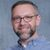# QlikView App Dev

Discussion Board for collaboration related to QlikView App Development.

Announcements
Skip the ticket, Chat with Qlik Support instead for instant assistance.
cancel
Showing results for
Did you mean:Creator III

## Using variable from input box in expression

Hi

I have an input box where I pick values from a list for variable vMargin.

I now want to use this variable in a simple expression in a chart, I'm getting a null value.

If i just use the expression =\$(vMargin), I get the value of variable as picked in the input box.

However, when I add another element to the margin, even a constant e.g. 100/\$(vMargin), I'm getting a null value.

Any ideas?

Thanks

Joe

1 Solution

Accepted SolutionsContributor III

I was able to get this to work using sample data if this is what you were going for:

sum({<Dim={'ValueA'}>} fact)/(1-\$(vMargin))

5 RepliesContributor III

try adding sum or aggr such as sum(100/\$(vMargin)) or aggr(100/\$(vMargin), Dim)Creator III
Author

Thanks Justin

The actual expression I have uses a Set Analysis expression as the numerator, and then the variable as the denominator.

So Sum({<Dim={'ValueA'}>}/(1-\$(vMargin))Contributor III

I was able to get this to work using sample data if this is what you were going for:

sum({<Dim={'ValueA'}>} fact)/(1-\$(vMargin))Creator III
Author

Thanks Justin

That works fine for me now.

Regards

JoeContributor III